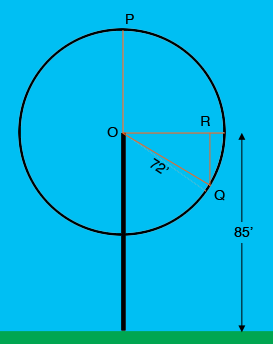SEARCH HOMEMath Central Quandaries & QueriesQuestion from Claire, a student: A Ferris wheel has a radius of 72 feet and its center is 85 feet above the ground. At the top of the Ferris wheel, Sam is in the topmost car. If the Ferris wheel makes two complete revolutions per minute, how high above the ground will Sam be after 10 seconds? A) 121 ft B) 108 ft C) 49 ft D) 36 ftHi Claire,

If the Ferris wheel makes two revolutions in a minute it makes one revolution in 30 seconds and hence one-third of a revolution in 10 seconds. One-third of $360^o$ is $120^o$ and in my diagram I have the Ferris wheel going clockwise so Sam is at the point Q after 10 secondsThe measure of the angle $ROQ$ is $120^o - 90^o = 30^o$. What is the length of $RQ?$ Subtract that from 85 feet to obtain Sam's height above the ground.

PennyMath Central is supported by the University of Regina and The Pacific Institute for the Mathematical Sciences.